13.7 Balance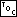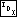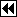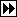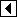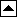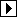We can balance a PBST in the same way that we would balance a BST without parent pointers. In fact, we'll use the same code, with the only change omitting only the maximum height check. This code doesn't set parent pointers, so afterward we traverse the tree to take care of that.

Here are the pieces of the core code that need to be repeated:

```511. <PBST balance function 511> =
<BST to vine function; bst => pbst 89>
<Vine to balanced PBST function 512>
<Update parent pointers function 514>

void pbst_balance (struct pbst_table *tree) {
assert (tree != NULL);

tree_to_vine (tree);
vine_to_tree (tree);
update_parents (tree);
}
```

This code is included in 489.

```512. <Vine to balanced PBST function 512> =
<BST compression function; bst => pbst 95>

static void vine_to_tree (struct pbst_table *tree) {
unsigned long vine;      /* Number of nodes in main vine. */
unsigned long leaves;    /* Nodes in incomplete bottom level, if any. */
int height;              /* Height of produced balanced tree. */

<Calculate leaves; bst => pbst 91>
<Reduce vine general case to special case; bst => pbst 92>
<Make special case vine into balanced tree and count height; bst => pbst 93>
}
```

This code is included in 511.

```513. <PBST extra function prototypes 513> =

/* Special PBST functions. */
void pbst_balance (struct pbst_table *tree);
```
##### Updating Parent Pointers

The procedure for rebalancing a binary tree leaves the nodes' parent pointers pointing every which way. Now we'll fix them. Incidentally, this is a general procedure, so the same code could be used in other situations where we have a tree to which we want to add parent pointers.

The procedure takes the same form as an inorder traversal, except that there is nothing to do in the place where we would normally visit the node. Instead, every time we move down to the left or the right, we set the parent pointer of the node we move to.

The code is straightforward enough. The basic strategy is to always move down to the left when possible; otherwise, move down to the right if possible; otherwise, repeatedly move up until we've moved up to the left to arrive at a node with a right child, then move to that right child.

```514. <Update parent pointers function 514> =
static void update_parents (struct pbst_table *tree) {
struct pbst_node *p;

if (tree->pbst_root == NULL)
return;

tree->pbst_root->pbst_parent = NULL;
for (p = tree->pbst_root; ; p = p->pbst_link)     {
for (; p->pbst_link != NULL; p = p->pbst_link)

for (; p->pbst_link == NULL; p = p->pbst_parent)         {
for (;;)             {
if (p->pbst_parent == NULL)
return;

if (p == p->pbst_parent->pbst_link)
break;
p = p->pbst_parent;
}
}